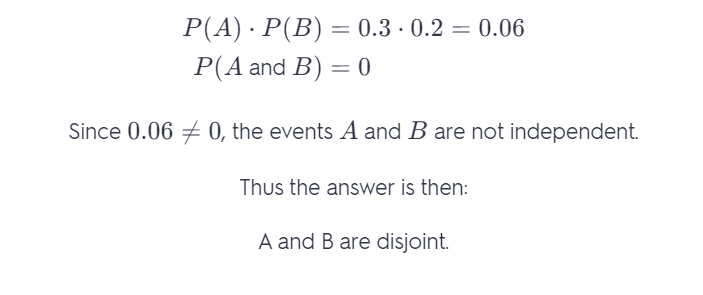# Given The Following: P(A) =0.3 P(B) = 0.2 P(A And B) = 0.4 Which Of The Following Is True?

We thoroughly check each answer to a question to provide you with the most correct answers. Found a mistake? Let us know about it through the REPORT button at the bottom of the page.

Given the following:

P(A) = 0.3

P(B) = 0.2

P(A or B) = 0.5

P(A and B) = 0

Which of the following is true?

A and B are disjoint.

A and B are neither disjoint nor independent.

A and B are independent.

A and B are disjoint and independent.

Answer: A and B are disjoint.

## Explanation

Two events are disjoint if the events cannot occur at the same time. Since P(A and B) = 0, the events A and B cannot occur at the same time, and thus events A and B are disjoint.

Two events are Independent if the probability that one event occurs in no way affects the probability of the other event occurring. Moreover, two events are independent if and only if P (A and B) = P(A) * P(B).